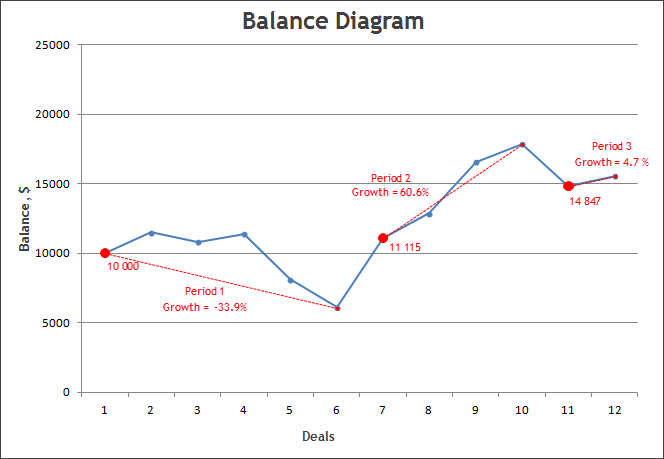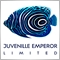# miscalculating grow per month balance account.34

Hi. I want calculate manually the grow per month of an account that I'm monitoring. But this value is different from the value reported in the website's information.

For example, I calculate a grow in September of 26.77%, but in the website this value is 31.38%.

What I'm doing wrong? I just divide the final balance and the initial balance, avoiding deposits and withdrawals, like the instructions showed here below:

How is the Growth in Signals Calculated?
The growth shows how the balance of an account grows. It is calculated so that the influence of deposits and withdrawals is avoided.

The entire trade history of an account is divided into periods between balance operations (deposits and withdrawals). First, the total growth coefficient (K) is calculated by multiplying the growth coefficients computed for each period between the balance operations (BO) and then the growth in percentage terms is calculated.

Growth Coefficient К = (Balance before BO1/Initial Deposit) * (Balance before BO2/Balance after BO1 * ... * Balance before BOn/Balance after BOn-1)

Growth in Percentage Terms = (К - 1) * 100%

On the chart below, the balance operations are marked with big red dots and the dashed lines indicate the periods of growth calculation:In this case, the total growth for the account is calculated as follows:

Growth Coefficient К = К1 * K2 * K3 = (6 615/10 000 * 17 847/11 115 * 15 547/14 847) = 1.1

Growth in Percentage Terms = (K-1) * 100% = (1.1 - 1) * 100 = 10%

Despite the current balance is about 50% higher than the initial deposit, the real growth due to trade operations is only 10%.Moderator
14170

Catbug1618:

Hi. I want calculate manually the grow per month of an account that I'm monitoring. But this value is different from the value reported in the website's information.

For example, I calculate a grow in September of 26.77%, but in the website this value is 31.38%.

What I'm doing wrong? I just divide the final balance and the initial balance, avoiding deposits and withdrawals, like the instructions showed here below:

How is the Growth in Signals Calculated?
The growth shows how the balance of an account grows. It is calculated so that the influence of deposits and withdrawals is avoided.

The entire trade history of an account is divided into periods between balance operations (deposits and withdrawals). First, the total growth coefficient (K) is calculated by multiplying the growth coefficients computed for each period between the balance operations (BO) and then the growth in percentage terms is calculated.

Growth Coefficient К = (Balance before BO1/Initial Deposit) * (Balance before BO2/Balance after BO1 * ... * Balance before BOn/Balance after BOn-1)

Growth in Percentage Terms = (К - 1) * 100%

On the chart below, the balance operations are marked with big red dots and the dashed lines indicate the periods of growth calculation:

In this case, the total growth for the account is calculated as follows:

Growth Coefficient К = К1 * K2 * K3 = (6 615/10 000 * 17 847/11 115 * 15 547/14 847) = 1.1

Growth in Percentage Terms = (K-1) * 100% = (1.1 - 1) * 100 = 10%

Despite the current balance is about 50% higher than the initial deposit, the real growth due to trade operations is only 10%.

You forget the compounding factor.

5% then 5% and then another 5% is not 15%, but 5% on top of 5% on top of 5%.34

Eleni Anna Branou:

You forget the compounding factor.

5% then 5% and then another 5% is not 15%, but 5% on top of 5% on top of 5%.

the compounding factor is applied when exist a deposit or withdrawal between trades in a month.I just see a deposit of 100 usd at the beginning of September, then 2 trades. That's all for September. I must applied the compounding factor in this case?Moderator
14170

Catbug1618:

the compounding factor is applied when exist a deposit or withdrawal between trades in a month.

I just see a deposit of 100 usd at the beginning of September, then 2 trades. That's all for September. I must applied the compounding factor in this case?

The compounding factor is always in force and has nothing to do with deposits or withdrawals.

Each new profit is calculated on top of all previous growth.34

Eleni Anna Branou:

The compounding factor is always in force and has nothing to do with deposits or withdrawals.

Each new profit is calculated on top of all previous growth.

Ok thank you!